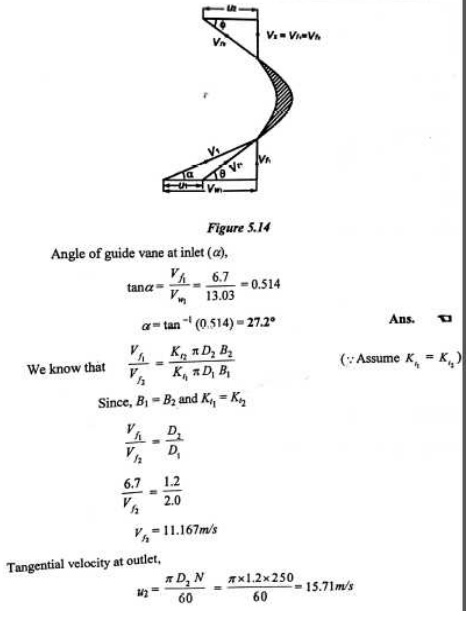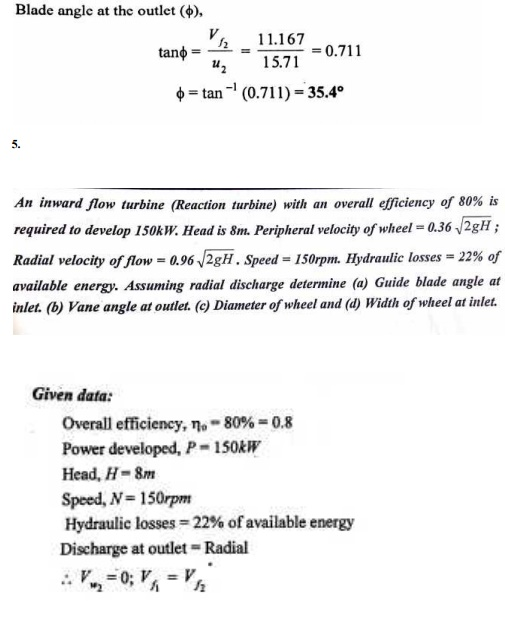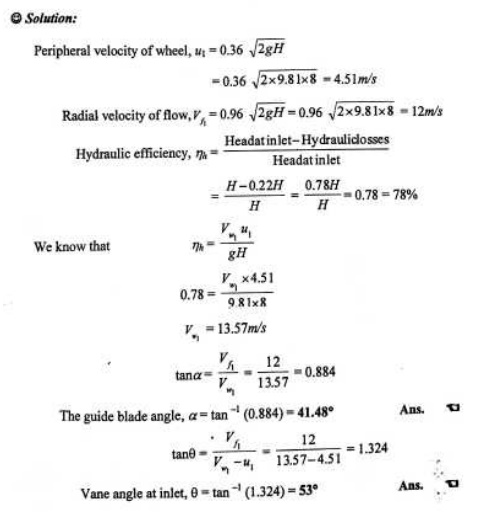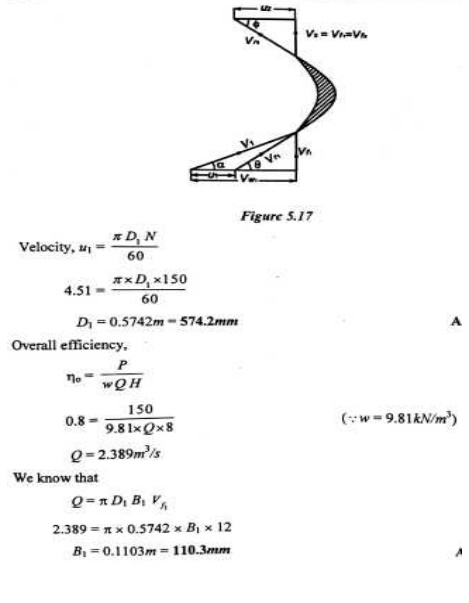Home | | Fluid Mechanics and Machinery | Important Answers and Solved Problems: Fluid Mechanics - Turbines

# Important Answers and Solved Problems: Fluid Mechanics - Turbines

Mechanical - Fluid Mechanics And Machinery - Turbines

TURBINES

1.  Define hydraulic machines.

Hydraulic machines which convert the energy of flowing water into mechanical energy.

3.   What is impulse turbine? Give example.

In impulse turbine all the energy converted into kinetic energy. From these the turbine will develop high kinetic energy power. This turbine is called impulse turbine. Example: Pelton turbine

4.  What is reaction turbine? Give example.

In a reaction turbine, the runner utilizes both potential and kinetic energies. Here portion of potential energy is converted into kinetic energy before entering into the turbine.

Example: Francis and Kaplan turbine.

5.  What is axial flow turbine?

In axial flow turbine water flows parallel to the axis of the turbine shaft. Example: Kaplan turbine

6.  What is mixed flow turbine?

In mixed flow water enters the blades radially and comes out axially, parallel to the turbine shaft. Example: Modern Francis turbine.

7.  What is the function of spear and nozzle?

The nozzle is used to convert whole hydraulic energy into kinetic energy. Thus the nozzle delivers high speed jet. To regulate the water flow through the nozzle and to obtain a good jet of water spear or nozzle is arranged.

Gross Head: The gross head is the difference between the water level at the reservoir and the level at the tailstock.

9.  Define hydraulic efficiency.

It is defined as the ratio of power developed by the runner to the power supplied by the water

jet.

10. Define mechanical efficiency.

It is defined as the ratio of power available at the turbine shaft to the power developed by the turbine runner.

11. Define volumetric efficiency.

It is defied as the volume of water actually striking the buckets to the total water supplied by the jet.

12. Define over all efficiency.

It is defined as the ratio of power available at the turbine shaft to the power available from the water jet.

13. Define the terms

(a) Hydraulic machines (b) Turbines (c) Pumps. a. Hydraulic machines:

Hydraulic machines are defined as those machines which convert either hydraulic energy into mechanical energy or mechanical energy into hydraulic energy.

b. Turbines;

The hydraulic machines which convert hydraulic energy into mechanical energy are called turbines.

c. Pumps:

The hydraulic Machines which convert mechanical energy into hydraulic energy are called

pumps.

14. What do you mean by gross head?

The difference between the head race level and tail race level when no water is flowing is known as gross head. It is denoted by Hg.

15. What do you mean by net head?

Net head is also known as effective head and is defined as the head available at the inlet of te turbine. It is denoted as H

16. What is draft tube? why it is used in reaction turbine?

The pressure at exit of runner of a reaction turbine is generally less than the atmospheric pressure. The water at exit cannot be directly discharged to tail race. A tube or pipe of gradually increasing area is used for discharging water from exit of turbine to tail race. This tube of increasing area is called draft tube.

17. What is the significance of specific speed?

Specific speed plays an important role for selecting the type of turbine. Also the performance of turbine can be predicted by knowing the specific speed of turbine.

18.. What are unit quantities?

Unit quantities are the quantities which are obtained when the head on the turbine is unity. They are unit speed, unit power unit discharge.

19. Why unit quantities are important

If a turbine is working under different heads, the behavior of turbine can be easily known from the values of unit quantities.

20. What do you understand by characteristic curves of turbine?

Characteristic curves of a hydraulic turbine are the curves, with the help of which the exact behavior and performance of turbine under different working conditions can be known.

21. Define the term 'governing of turbine'.

Governing of turbine is defined as the operation by which the speed of the turbine is kept constant under all conditions of working. It is done by oil pressure governor.

22. What are the types of draft tubes?

The following are the important types of draft tubes which are commonly used.

a. Conical draft tubes

b. Simple elbow tubes

d.Elbow draft tubes with circular inlet and rectangular outlet.Study Material, Lecturing Notes, Assignment, Reference, Wiki description explanation, brief detail
Mechanical : Fluid Mechanics And Machinery : Turbines : Important Answers and Solved Problems: Fluid Mechanics - Turbines |

Related Topics